﻿ 基于扩散过程核权r阶幂变差瞬时波动率估计的VaR度量 Measurement of Value at Risk Based on Kernel-Weighted r-Power Variation Estimation of Instantaneous Volatility for Stochastic Diffusion Model

Statistics and Application
Vol. 08  No. 06 ( 2019 ), Article ID: 33307 , 13 pages
10.12677/SA.2019.86103

Measurement of Value at Risk Based on Kernel-Weighted r-Power Variation Estimation of Instantaneous Volatility for Stochastic Diffusion Model

Jiawei Su, Ruixin Wei, Yiyao Liu

School of Mathematics and Statistics, Guangxi Normal University, Guilin GuangxiReceived: Nov. 15th, 2019; accepted: Nov. 28th, 2019; published: Dec. 5th, 2019ABSTRACT

The risk of financial assets is related to the volatility of return in the financial market. Therefore, when we estimate VaR, which serves as a widely used measurement of assets’ risk, with parametric method, volatility is one of the most important input variables. In this paper, we proposed kernel-weighted estimators of instantaneous volatility with r (r > 0)-power variation for stochastic diffusion model, proving its asymptotic unbiasedness. A simulation study will examine the finite sample properties of the VaR estimated by the estimators we proposed. It is shown that the VaR we estimated passed the test of significance, which means it is reliable to be the measurement of assets’ risk.

Keywords:VaR, Instantaneous Volatility, Stochastic Diffusion Model1. 引言

2. 基本原理和假设

2.1. 模型设定及估计量的定义

$\text{d}{X}_{t}={\mu }_{t}\text{d}t+{\sigma }_{t}\text{d}{W}_{t}$ (2.1)

${\stackrel{^}{\sigma }}_{\tau }^{r}={k}_{r}^{-1}{\delta }^{1-r/2}\underset{i=1}{\overset{n}{\sum }}\text{ }\text{ }{K}_{h}\left({t}_{i}-\tau \right){|\Delta {X}_{{t}_{i}}|}^{r},$ (2.2)

2.2. 基本假设

(A1) 过程 $\left\{{\mu }_{t}\right\}$$\left\{{\sigma }_{t}\right\}$ 都与过程 $\left\{{W}_{t}\right\}$ 独立，且在 $t\in \left[0,T\right]$ 上可积和有界。

(A2) 存在 $\lambda >0,C>0$，使得对于任意 $t,s\in \left[0,T\right]$$r>0$，有

$|{\sigma }_{t}^{r}-{\sigma }_{s}^{r}|\le C{|t-s|}^{\lambda }.$

(A3) (i) 核函数K(u)是有界概率密度函数。对于λ > 0，有 ${\int }_{-\infty }^{\infty }{|u|}^{\lambda }K\left(u\right)\text{d}u<\infty$。(ii) 存在 $\beta >0,C>0$，使得对于任意 $u,v\in R$，有

$|K\left(u\right)-K\left(v\right)|\le C{|u-v|}^{\beta }.$

(A4) 当 $n\to \infty$ 时，有 $h\to 0$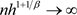$\Delta {X}_{{t}_{i}}={\int }_{{t}_{i-1}}^{{t}_{i}}\text{ }\text{ }{\mu }_{t}\text{d}t+{\int }_{{t}_{i-1}}^{{t}_{i}}\text{ }\text{ }{\sigma }_{t}\text{d}{W}_{t}.$ (2.3)

$\Delta {\stackrel{˜}{X}}_{{t}_{i}}={\int }_{{t}_{i-1}}^{{t}_{i}}\text{ }\text{ }{\sigma }_{s}\text{d}{W}_{s},$ (2.4)

$\stackrel{˜}{{\stackrel{^}{\sigma }}_{\tau }^{r}}={\kappa }_{r}^{-1}{\Delta }_{n}^{1-r/2}\underset{i=1}{\overset{n}{\sum }}\text{ }\text{ }{K}_{h}\left({t}_{i}-\tau \right){|\Delta {\stackrel{˜}{X}}_{{t}_{i}}|}^{r}.$ (2.5)

$\stackrel{˜}{{\stackrel{^}{\sigma }}_{\tau }^{r}}={\kappa }_{r}^{-1}{\Delta }_{n}^{1-r/2}\underset{i=1}{\overset{n}{\sum }}\text{ }\text{ }{K}_{h}\left({t}_{i}-\tau \right){|{\int }_{{t}_{i-1}}^{{t}_{i}}\text{ }{\sigma }_{t}\text{d}{W}_{t}|}^{r},$ (2.6)

${\stackrel{^}{\sigma }}_{\tau }^{r}={\kappa }_{r}^{-1}{\Delta }_{n}^{1-r/2}\underset{i=1}{\overset{n}{\sum }}\text{ }\text{ }{K}_{h}\left({t}_{i}-\tau \right){|{\int }_{{t}_{i-1}}^{{t}_{i}}\text{ }{\mu }_{t}\text{d}t+{\int }_{{t}_{i-1}}^{{t}_{i}}\text{ }{\sigma }_{t}\text{d}{W}_{t}|}^{r}.$ (2.7)

${\int }_{{t}_{i-1}}^{{t}_{i}}\text{ }\text{ }{\sigma }_{s}\text{d}{W}_{s}\stackrel{law}{=}{\left({\int }_{{t}_{i-1}}^{{t}_{i}}\text{ }{\sigma }_{s}^{2}\text{d}s\right)}^{1/2}{U}_{i},$ (2.8)

${v}_{ni}\left(\tau \right)={K}_{h}\left({t}_{i}-\tau \right)\text{d}s{\left({\int }_{{t}_{i-1}}^{{t}_{i}}\text{ }{\sigma }_{s}^{2}\text{d}s\right)}^{r/2},$ (2.9)

$\stackrel{˜}{{\stackrel{^}{\sigma }}_{\tau }^{r}}\stackrel{law}{=}{\kappa }_{r}^{-1}{\Delta }_{n}^{1-r/2}\underset{i=1}{\overset{n}{\sum }}\text{ }\text{ }{v}_{ni}\left(\tau \right){|{U}_{i}|}^{r}.$ (2.10)

3. 估计量的渐近无偏性

Theorem 1 在条件(A1)~(A4)同时满足的情况下，有

$E\left({\stackrel{^}{\sigma }}_{\tau }^{r}|\mu ,\sigma \right)-{\sigma }_{\tau }^{r}=O\left({n}^{-1}+{h}_{n}^{\lambda }+{\left(n{h}_{n}^{1+1/\beta }\right)}^{-\beta }\right),a.s..$ (3.1)

$\begin{array}{c}E\left[\left({\stackrel{^}{\sigma }}_{\tau }^{r}-{\sigma }_{\tau }^{r}\right)|{\mu }_{t},{\sigma }_{t}\right]=E\left[\left({\stackrel{^}{\sigma }}_{\tau }^{r}-\stackrel{˜}{{\stackrel{^}{\sigma }}_{\tau }^{r}}\right)|{\mu }_{t},{\sigma }_{t}\right]+E\left[\stackrel{˜}{{\stackrel{^}{\sigma }}_{\tau }^{r}}|{\sigma }_{t}\right]-{\sigma }_{\tau }^{r}\\ =O\left({n}^{-1}\right)+E\left[\stackrel{˜}{{\stackrel{^}{\sigma }}_{\tau }^{r}}|{\sigma }_{t}\right]-{\sigma }_{\tau }^{r},\text{\hspace{0.17em}}a.s..\end{array}$ (3.2)

${I}_{1n}\left(\tau \right)={\Delta }_{n}^{1-r/2}\underset{i=1}{\overset{n}{\sum }}\text{ }\text{ }{v}_{ni}\left(\tau \right)-{\int }_{0}^{T}\text{ }\text{ }{K}_{h}\left(s-\tau \right){\sigma }_{s}^{r}\text{d}s,$ (3.3)

${I}_{2n}\left(\tau \right)={\int }_{0}^{T}\text{ }\text{ }{K}_{h}\left(s-\tau \right){\sigma }_{s}^{r}\text{d}s-{\sigma }_{\tau }^{r},$ (3.4)

$E\left(\stackrel{˜}{{\stackrel{^}{\sigma }}_{\tau }^{r}}|{\sigma }_{t}\right)-{\sigma }_{\tau }^{r}={\Delta }_{n}^{1-r/2}\underset{i=1}{\overset{n}{\sum }}\text{ }\text{ }{v}_{ni}\left(\tau \right)-{\sigma }_{\tau }^{r}={I}_{1n}\left(\tau \right)+{I}_{2n}\left(\tau \right).$ (3.5)

${I}_{1n}\left(\tau \right)=O\left({n}^{-\lambda }+{\left(n{h}^{1+1/\beta }\right)}^{-\beta }\right),a.s.,$ (3.6)

$\begin{array}{l}|{\sigma }_{\tau }^{r}{\int }_{-\infty }^{-\tau /{h}_{n}}\text{ }\text{ }K\left(u\right)\text{d}u+{\sigma }_{\tau }^{r}{\int }_{\left(T-\tau \right)/{h}_{n}}^{\infty }\text{ }\text{ }K\left(u\right)\text{d}u|\\ \le C{h}_{n}^{\lambda }{\int }_{-\infty }^{-\tau /{h}_{n}}{|u|}^{\lambda }|K\left(u\right)|\text{d}u+C{h}_{n}^{\lambda }{\int }_{\left(T-\tau \right)/{h}_{n}}^{\infty }{|u|}^{\lambda }|K\left(u\right)|\text{d}u\\ =O\left({h}_{n}^{\lambda }\right),\end{array}$ (3.7)

$\begin{array}{c}{I}_{2n}\left(\tau \right)={\int }_{0}^{T}\text{ }\text{ }{K}_{h}\left(s-\tau \right){\sigma }_{s}^{r}\text{d}s-{\sigma }_{\tau }^{r}{\int }_{\infty }^{\infty }\text{ }K\left(u\right)\text{d}u\\ ={\int }_{0}^{T}\text{ }\text{ }{K}_{h}\left(s-\tau \right){\sigma }_{s}^{r}\text{d}s-{\sigma }_{\tau }^{r}{\int }_{-\tau /{h}_{n}}^{\left(T-\tau \right)/{h}_{n}}\text{ }\text{ }K\left(u\right)\text{d}u+O\left({h}_{n}^{\lambda }\right)\\ ={\int }_{0}^{T}\text{ }\text{ }{K}_{h}\left(s-\tau \right){\sigma }_{s}^{r}\text{d}s-{\sigma }_{\tau }^{r}{\int }_{0}^{T}\text{ }\text{ }{K}_{h}\left(s-\tau \right)\text{d}s+O\left({h}_{n}^{\lambda }\right)\\ ={\int }_{0}^{T}\text{ }\text{ }{K}_{h}\left(s-\tau \right)\left({\sigma }_{s}^{r}-{\sigma }_{\tau }^{r}\right)\text{d}s+O\left({h}_{n}^{\lambda }\right)\\ ={\int }_{-\tau /{h}_{n}}^{\left(T-\tau \right)/{h}_{n}}\text{ }\text{ }K\left(u\right)\left({\sigma }_{\tau +u{h}_{n}}^{r}-{\sigma }_{\tau }^{r}\right)\text{d}u+O\left({h}_{n}^{\lambda }\right).\end{array}$ (3.8)

$\begin{array}{c}|{I}_{2n}\left(\tau \right)|\le {\int }_{-\tau /{h}_{n}}^{\left(T-\tau \right)/{h}_{n}}|K\left(u\right)||{\sigma }_{\tau +u{h}_{n}}^{r}-{\sigma }_{\tau }^{r}|\text{d}u+O\left({h}_{n}^{\lambda }\right)\\ \le C{h}_{n}^{\lambda }{\int }_{-\tau /{h}_{n}}^{\left(T-\tau \right)/{h}_{n}}{|u|}^{\lambda }|K\left(u\right)|\text{d}u+O\left({h}_{n}^{\lambda }\right)\\ =O\left({h}_{n}^{\lambda }\right),a.s..\end{array}$ (3.9)

${I}_{2n}\left(\tau \right)=O\left({h}_{n}^{\lambda }\right),a.s..$ (3.10)

$E\left(\stackrel{˜}{{\stackrel{^}{\sigma }}_{\tau }^{r}}|{\sigma }_{t}\right)-{\sigma }_{\tau }^{r}=O\left({h}_{n}^{\lambda }+{\left(n{h}_{n}^{1+1/\beta }\right)}^{-\beta }\right),a.s..$ (3.11)

4. 数值模拟

4.1. 数据的选取

$\text{d}{X}_{t}={\mu }_{t}\text{d}t+{\sigma }_{t}\text{d}{W}_{1,t},$ (4.1)

$\text{d}{\sigma }_{t}^{2}=\beta \left(\alpha -{\sigma }_{t}^{2}\right)\text{d}t+\kappa {\sigma }_{t}^{2}\text{d}{W}_{2,t},$ (4.2)

$\Delta {X}_{i\delta }={\mu }_{t}\delta +{\sigma }_{\left(i-1\right)\delta }\sqrt{\delta }{\epsilon }_{1,i},$ (4.3)

$\Delta {\sigma }_{i\delta }^{2}=\beta \left(\alpha -{\sigma }_{\left(i-1\right)\delta }^{2}\right)\delta +\kappa {\sigma }_{\left(i-1\right)\delta }^{2}\sqrt{\delta }{\epsilon }_{2,i},$ (4.4)

(1) 依据离散模型(4.4)获取真值 $\left\{{\sigma }_{t}\right\}$。因离散模型(4.4)在提取间隔尽可能小的时候才会近似于连续模型(4.2)，所以在离散模型(4.4)中我们取时间间隔 $\delta =\Delta /100$，其中 ${\Delta }^{-1}=3×60×24×2$

(2) 依据离散模型(4.3)以20秒为提取间隔来产生样本 $\left\{\Delta {X}_{i\delta }\right\}$。离散模型(4.3)中与20秒提取间隔对应的抽样频率为 ${\delta }^{-1}=3×60×24×2$

(3) 选取核函数和窗宽。本文选用高斯核来作为核估计中的核函数，而窗宽则依照无偏最小平方交叉实证法来选取。

(4) 取 $r=1$，根据公式

${\stackrel{^}{\sigma }}_{\tau }^{r}={k}_{r}^{-1}{\delta }^{1-r/2}\underset{i=1}{\overset{n}{\sum }}\text{ }\text{ }{K}_{h}\left({t}_{i}-\tau \right){|\Delta {X}_{{t}_{i}}|}^{r},$ (4.5)

4.2. 数据的基本统计特征及正态性检验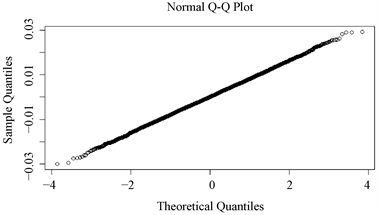Figure 1. Q-Q plot of the log-return sequence

4.3. 数据的平稳性检验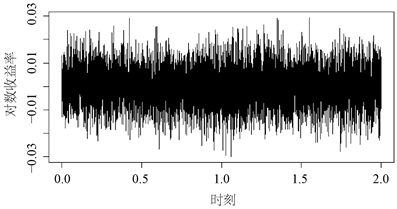Figure 2. Sequence diagram of the log-returnTable 2. ADF test result of the log-return based on simulated data

4.4. 数据的自相关性检验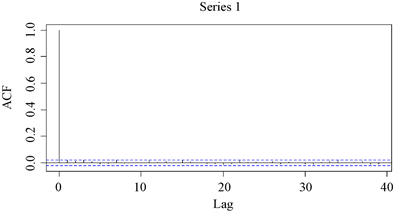Figure 3. Autocorrelogram of the log-return based on simulated data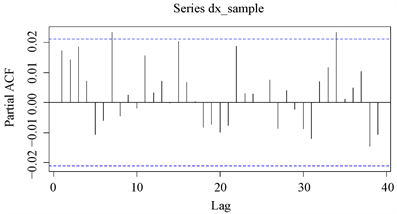Figure 4. Partial autocorrelogram of the log-return based on simulated data

4.5. 数据的异方差检验Table 3. ARCH-LM test result of the log-return based on simulated data

4.6. VaR的定义及计算

VaR通常可理解为“给定某置信水平，投资组合在一定持有期内的最大损失”。设 ${X}_{{t}_{i}}$ 为投资组合在时刻 ${t}_{i}$ 时的对数价格，则该投资组合在持有期 $\delta$ 内的收益率为

$\Delta {X}_{{t}_{i}}={X}_{{t}_{i}}-{X}_{{t}_{i-1}}$ (4.6)

$VaR={v}_{o}{z}_{\alpha }\sigma \sqrt{\delta }$ (4.7)

${\stackrel{^}{\sigma }}_{\tau }^{r}={\kappa }_{r}^{-1}{\Delta }_{n}^{1-r/2}\underset{i=1}{\overset{n}{\sum }}\text{ }\text{ }{K}_{h}\left({t}_{i}-\tau \right){|\Delta {X}_{{t}_{i}}|}^{r}.$ (4.8)

$r=1$，将估计值 ${\stackrel{^}{\sigma }}_{\tau }^{r}$ 代入(4.7)中，即可得到 $\left\{Va{R}_{\tau },\tau \in \left[0,T\right]\right\}$ 序列

$Va{R}_{\tau }={v}_{o}{z}_{\alpha }{\stackrel{^}{\sigma }}_{\tau }\sqrt{\delta }$ (4.9)

4.7. VaR的有效性检验

$LR=-2\mathrm{ln}\left({\left(1-\alpha \right)}^{T-N}{\alpha }^{N}\right)+2\mathrm{ln}\left({\left(1-N/T\right)}^{T-N}{\left(N/T\right)}^{N}\right)$ (4.10)Table 5. The result of the Kupiec test under the significance level of 5%

5. 结论

Measurement of Value at Risk Based on Kernel-Weighted r-Power Variation Estimation of Instantaneous Volatility for Stochastic Diffusion Model[J]. 统计学与应用, 2019, 08(06): 909-921. https://doi.org/10.12677/SA.2019.86103

1. 1. Fan, J.Q. and Wang, Y.Z. (2008) Spot Volatility Estimation for High-Frequency Data. Statistics and Its Interface, 1, 279-288. https://doi.org/10.4310/SII.2008.v1.n2.a5

2. 2. Kristensen, D. (2010) Nonparametric Filtering of the Realized Spot Volatility: A Kernel Based Approach. Econometric Theory, 26, 60-93. https://doi.org/10.1017/S0266466609090616

3. 3. Ait-Sahalia, Y., Mykland, P.A. and Zhang, L. (2010) Ultra High Frequency Volatility Estimation with Dependent Microstructure Noise. Journal of Econometrics, 160, 160-175. https://doi.org/10.1016/j.jeconom.2010.03.028

4. 4. Sabel, T., Schmidt-Hieber, J. and Munk, A. (2015) Spot Volatility Estimation for High-Frequency Data: Adaptive Estimation in Practice. In: Modelling and Stochastic Learning for Forecasting in High Dimensions, 213-241. https://doi.org/10.1007/978-3-319-18732-7_12

5. 5. Vatter, T., Wu, H., Chavezdemoulin, V. and Yu, B. (2015) Non-Parametric Estimation of Intraday Spot Volatility: Disentangling Instantaneous Trend and Seasonality. Econometrics, 3, 864-887. https://doi.org/10.3390/econometrics3040864

6. 6. 蔡井伟, 陈萍, 梅霞. 基于两步平滑的瞬时波动率非参数估计[J]. 统计与决策, 2017(23): 88-90.

7. 7. 刘红月. 基于高频低频数据相结合的瞬时波动率分布估计[D]: [硕士学位论文]. 苏州: 苏州大学, 2017.

8. 8. 王江涛, 周勇. 高频数据波动率非参数估计及窗宽选择[J]. 系统工程理论与实践, 2018, 38(10): 2491-2500.

Lemma 6.1. 记

${\gamma }_{i}={\Delta }_{n}^{-1}{\int }_{{t}_{i-1}}^{{t}_{i}}\text{ }{\mu }_{s}\text{d}s,\text{\hspace{0.17em}}\text{\hspace{0.17em}}\text{\hspace{0.17em}}{\theta }_{i}={\Delta }_{n}^{-1}{\int }_{{t}_{i-1}}^{{t}_{i}}\text{ }{\sigma }_{s}^{2}\text{d}s,\text{\hspace{0.17em}}\text{\hspace{0.17em}}\text{\hspace{0.17em}}{\rho }_{i}={\theta }_{i}^{-1/2}{\gamma }_{i},$ (6.1)

$h\left({U}_{i}\right)={|{\rho }_{i}{\Delta }_{n}^{1/2}+{U}_{i}|}^{r}-{|{U}_{i}|}^{r}.$ (6.2)

$E\left[h\left({U}_{i}\right)|{\mu }_{t},{\sigma }_{t}\right]=O\left({\Delta }_{n}\right),\text{\hspace{0.17em}}a.s.,$ (6.3)

Proof 由条件(A1)和积分中值定理得，存在常数 ${C}_{1}>0$${C}_{2}>0$，使得 ${C}_{1}\le {\mathrm{max}}_{i}|{\theta }_{i}|\le {C}_{2}$ 以及 ${\mathrm{max}}_{i}|{\gamma }_{i}|\le {C}_{2}$，从而有 $|{\rho }_{i}|\le C<\infty$

$\begin{array}{c}E\left[h\left({U}_{i}\right)|{\mu }_{t},{\sigma }_{t}\right]=E\left[{|{\rho }_{i}{\Delta }_{n}^{1/2}+{U}_{i}|}^{r}|{\mu }_{t},{\sigma }_{t}\right]-E\left[{|{U}_{i}|}^{r}\right]\\ =\frac{1}{\sqrt{2\pi }}{\int }_{-\infty }^{\infty }{|{\rho }_{i}{\Delta }_{n}^{1/2}+x|}^{r}{\text{e}}^{-{x}^{2}/2}\text{d}x-{\kappa }_{r}.\end{array}$ (6.4)

$u={\rho }_{i}{\Delta }_{n}^{1/2}+x$，那么有(6.5)

$E\left[h\left({U}_{i}\right)|{\mu }_{t},{\sigma }_{t}\right]=\frac{1}{\sqrt{2\pi }}\left(1+O\left({\Delta }_{n}\right)\right){\int }_{-\infty }^{\infty }{|u|}^{r}{\text{e}}^{-{u}^{2}/2}{\text{e}}^{u{\rho }_{i}{\Delta }_{n}^{1/2}}\text{d}u-{\kappa }_{r},$ (6.6)

$\frac{1}{\sqrt{2\pi }}{\int }_{-\infty }^{\infty }{|u|}^{r}{\text{e}}^{-{u}^{2}/2}{\text{e}}^{u{\rho }_{i}{\Delta }_{n}^{1/2}}\text{d}u=\frac{{\text{e}}^{{\rho }_{i}^{2}{\Delta }_{n}}}{\sqrt{2\pi }}{\int }_{-\infty }^{\infty }{|u|}^{r}{\text{e}}^{-{\left(u-{\rho }_{i}{\Delta }_{n}^{1/2}\right)}^{2}/2}\text{d}u\le C<\infty .$ (6.7)

$\begin{array}{l}E\left[h\left({U}_{i}\right)|{\mu }_{t},{\sigma }_{t}\right]\\ =\frac{1}{\sqrt{2\pi }}{\int }_{-\infty }^{\infty }{|u|}^{r}{\text{e}}^{-{u}^{2}/2}{\text{e}}^{u{\rho }_{i}{\Delta }_{n}^{1/2}}\text{d}u-{\kappa }_{r}+O\left(\Delta \right),\text{\hspace{0.17em}}a.s..\end{array}$ (6.8)

$\begin{array}{l}\frac{1}{\sqrt{2\pi }}{\int }_{-\infty }^{\infty }{|u|}^{r}{\text{e}}^{-{u}^{2}/2}{\text{e}}^{u{\rho }_{i}{\Delta }_{n}^{1/2}}\text{d}u\\ =\frac{1}{\sqrt{2\pi }}{\int }_{-\infty }^{\infty }{|u|}^{r}{\text{e}}^{-{u}^{2}/2}\left\{1+u{\rho }_{i}{\Delta }_{n}^{1/2}+\frac{{u}^{2}{\left({\rho }_{i}{\Delta }_{n}^{1/2}\right)}^{2}}{2!}+\frac{{u}^{3}{\left({\rho }_{i}{\Delta }_{n}^{1/2}\right)}^{3}}{3!}+\cdots \right\}\text{d}u\\ =\frac{1}{\sqrt{2\pi }}{\int }_{-\infty }^{\infty }{|u|}^{r}{\text{e}}^{-{u}^{2}/2}\left\{1+\frac{{u}^{2}{\left({\rho }_{i}{\Delta }_{n}^{1/2}\right)}^{2}}{2!}+\frac{{u}^{4}{\left({\rho }_{i}{\Delta }_{n}^{1/2}\right)}^{4}}{4!}+\frac{{u}^{6}{\left({\rho }_{i}{\Delta }_{n}^{1/2}\right)}^{6}}{6!}+\cdots \right\}\text{d}u\\ ={\kappa }_{r}+\frac{1}{\sqrt{2\pi }}{\int }_{-\infty }^{\infty }{|u|}^{r}{\text{e}}^{-{u}^{2}/2}\left\{\frac{{u}^{2}{\left({\rho }_{i}{\Delta }_{n}^{1/2}\right)}^{2}}{2!}+\frac{{u}^{4}{\left({\rho }_{i}{\Delta }_{n}^{1/2}\right)}^{4}}{4!}+\frac{{u}^{6}{\left({\rho }_{i}{\Delta }_{n}^{1/2}\right)}^{6}}{6!}+\cdots \right\}\text{d}u\\ ={\kappa }_{r}+{\left({\rho }_{i}{\Delta }_{n}^{1/2}\right)}^{2}\frac{1}{\sqrt{2\pi }}{\int }_{-\infty }^{\infty }{|u|}^{r+2}{\text{e}}^{-{u}^{2}/2}\left\{\frac{1}{2!}+\frac{{u}^{4}{\left({\rho }_{i}{\Delta }_{n}^{1/2}\right)}^{4}}{4!}+\frac{{u}^{6}{\left({\rho }_{i}{\Delta }_{n}^{1/2}\right)}^{6}}{6!}+\cdots \right\}\text{d}u,\end{array}$ (6.9)(6.10)

Lemma 6.2 若条件(A3)和(A4)同时满足，那么对于任意 $\tau \in \left[0,T\right]$，有

${\Delta }_{n}\underset{i=1}{\overset{n}{\sum }}\text{ }\text{ }{K}_{h}\left({t}_{i}-\tau \right)={\int }_{-\infty }^{\infty }\text{ }\text{ }K\left(u\right)\text{d}u+O\left({h}_{n}^{\lambda }+{\left(n{h}_{n}^{1+1/\beta }\right)}^{-\beta }\right).$ (6.11)

${\Delta }_{n}\underset{i=1}{\overset{n}{\sum }}\text{ }\text{ }{K}_{h}\left({t}_{i}-\tau \right)\le C.$ (6.12)

Proof 由积分中值定理得，存在 ${\stackrel{^}{t}}_{i}\in \left[{t}_{i-1},{t}_{i}\right]$，使得

$\underset{i=1}{\overset{n}{\sum }}{\int }_{{t}_{i-1}}^{{t}_{i}}\text{ }\text{ }{K}_{h}\left(s-\tau \right)\text{d}s={\Delta }_{n}\underset{i=1}{\overset{n}{\sum }}\text{ }\text{ }{K}_{h}\left({\stackrel{^}{t}}_{i}-\tau \right).$ (6.13)

$\begin{array}{l}\underset{i=1}{\overset{n}{\sum }}{\int }_{{t}_{i-1}}^{{t}_{i}}\text{ }\text{ }{K}_{h}\left(s-\tau \right)\text{d}s-{\int }_{-\infty }^{\infty }\text{ }\text{ }K\left(u\right)\text{d}u\\ ={\int }_{0}^{T}\text{ }\text{ }{K}_{h}\left(s-\tau \right)\text{d}s-{\int }_{-\infty }^{\infty }\text{ }\text{ }{K}_{h}\left(s-\tau \right)\text{d}s\\ =-{\int }_{-\infty }^{0}\text{ }\text{ }{K}_{h}\left(s-\tau \right)\text{d}s-{\int }_{T}^{\infty }\text{ }\text{ }{K}_{h}\left(s-\tau \right)\text{d}s,\end{array}$ (6.14)

$\begin{array}{l}|{\int }_{-\infty }^{0}\text{ }\text{ }{K}_{h}\left(s-\tau \right)\text{d}s+{\int }_{T}^{\infty }\text{ }\text{ }{K}_{h}\left(s-\tau \right)\text{d}s|\\ =|{\int }_{-\infty }^{-\tau /h}\text{ }\text{ }K\left(u\right)\text{d}u+{\int }_{\left(T-\tau \right)/h}^{\infty }\text{ }\text{ }K\left(u\right)\text{d}u|\\ \le \frac{{h}^{\lambda }}{{\tau }^{\lambda }}{\int }_{-\infty }^{-\tau /h}{|u|}^{\lambda }K\left(u\right)\text{d}u+\frac{{h}^{\lambda }}{{\left(T-\tau \right)}^{\lambda }}{\int }_{\left(T-\tau \right)/h}^{\infty }{|u|}^{\lambda }K\left(u\right)\text{d}u\\ \le C\left(\frac{1}{{\tau }^{\lambda }}+\frac{1}{{\left(T-\tau \right)}^{\lambda }}\right){h}^{\lambda }.\end{array}$ (6.15)

$\underset{i=1}{\overset{n}{\sum }}{\int }_{{t}_{i-1}}^{{t}_{i}}\text{ }\text{ }{K}_{h}\left(s-\tau \right)\text{d}s={\int }_{-\infty }^{\infty }\text{ }\text{ }K\left(u\right)\text{d}u+O\left({h}^{\lambda }\right).$ (6.16)

$\begin{array}{l}{\Delta }_{n}\underset{i=1}{\overset{n}{\sum }}\text{ }\text{ }{K}_{h}\left({t}_{i}-\tau \right)\\ ={\Delta }_{n}\underset{i=1}{\overset{n}{\sum }}\text{ }\text{ }{K}_{h}\left({t}_{i}-\tau \right)-{\Delta }_{n}\underset{i=1}{\overset{n}{\sum }}\text{ }\text{ }{K}_{h}\left({\stackrel{^}{t}}_{i}-\tau \right)+\underset{i=1}{\overset{n}{\sum }}{\int }_{{t}_{i-1}}^{{t}_{i}}{K}_{h}\left(s-\tau \right)\text{d}s\\ \le {\Delta }_{n}\underset{i=1}{\overset{n}{\sum }}|{K}_{h}\left({t}_{i}-\tau \right)-{K}_{h}\left({\stackrel{^}{t}}_{i}-\tau \right)|+\underset{i=1}{\overset{n}{\sum }}{\int }_{{t}_{i-1}}^{{t}_{i}}{K}_{h}\left(s-\tau \right)\text{d}s\\ ={\Delta }_{n}\underset{i=1}{\overset{n}{\sum }}|{K}_{h}\left({t}_{i}-\tau \right)-{K}_{h}\left({\stackrel{^}{t}}_{i}-\tau \right)|+{\int }_{-\infty }^{\infty }\text{ }\text{ }K\left(u\right)\text{d}u+O\left({h}^{\lambda }\right)\\ ={\int }_{-\infty }^{\infty }\text{ }\text{ }K\left(u\right)\text{d}u+O\left({h}_{n}^{\lambda }+{\left(n{h}_{n}^{1+1/\beta }\right)}^{-\beta }\right).\end{array}$ (6.17)

Lemma 6.3 假设条件(A1)~(A4)同时满足，那么有

${\Delta }_{n}^{1-r/2}\underset{i=1}{\overset{n}{\sum }}\text{ }\text{ }{v}_{ni}\left(\tau \right)-{\int }_{0}^{T}\text{ }{K}_{h}\left(s-\tau \right){\sigma }_{s}^{r}\text{d}s=O\left({\left(\frac{1}{n}\right)}^{\lambda }+{\left(\frac{1}{n{h}^{1+1/\beta }}\right)}^{\beta }\right),\text{\hspace{0.17em}}a.s..$ (6.18)

Proof 由积分中值定理得，存在 ${\stackrel{^}{s}}_{i},{\stackrel{˜}{s}}_{i},{\theta }_{i}\in \left({t}_{i-1},{t}_{i}\right)\left(i=1,2,\cdots ,n\right)$，使得

$\begin{array}{l}|{\Delta }_{n}^{1-r/2}\underset{i=1}{\overset{n}{\sum }}\text{ }\text{ }{v}_{ni}\left(\tau \right)-{\int }_{0}^{T}\text{ }\text{ }{K}_{h}\left(s-\tau \right){\sigma }_{s}^{r}\text{d}s|\\ =|{\Delta }_{n}^{1-r/2}\underset{i=1}{\overset{n}{\sum }}\text{ }\text{ }{K}_{h}\left({t}_{i}-\tau \right){\left({\int }_{{t}_{i-1}}^{{t}_{i}}\text{ }\text{ }{\sigma }_{s}^{2}\text{d}s\right)}^{r/2}-\underset{i=1}{\overset{n}{\sum }}\text{ }\text{ }{K}_{h}\left({t}_{i}-\tau \right){\int }_{{t}_{i-1}}^{{t}_{i}}\text{ }\text{ }{\sigma }_{s}^{r}\text{d}s\\ \text{\hspace{0.17em}}\text{\hspace{0.17em}}\text{ }\text{ }+\underset{i=1}{\overset{n}{\sum }}\text{ }\text{ }{K}_{h}\left({t}_{i}-\tau \right){\int }_{{t}_{i-1}}^{{t}_{i}}\text{ }\text{ }{\sigma }_{s}^{r}\text{d}s-\underset{i=1}{\overset{n}{\sum }}{\int }_{{t}_{i-1}}^{{t}_{i}}\text{ }\text{ }{K}_{h}\left(s-\tau \right){\sigma }_{s}^{r}\text{d}s|\\ =|{\Delta }_{n}\underset{i=1}{\overset{n}{\sum }}\text{ }\text{ }{K}_{h}\left({t}_{i}-\tau \right)\left({\stackrel{^}{s}}_{i}^{r}-{\theta }_{i}^{r}\right)+\underset{i=1}{\overset{n}{\sum }}{\int }_{{t}_{i-1}}^{{t}_{i}}\left[{K}_{h}\left({t}_{i}-\tau \right)-{K}_{h}\left(s-\tau \right)\right]{\sigma }_{s}^{r}\text{d}s|\end{array}$

$\begin{array}{l}\le |{\Delta }_{n}\underset{i=1}{\overset{n}{\sum }}\text{ }\text{ }{K}_{h}\left({t}_{i}-\tau \right)|{\stackrel{^}{s}}_{i}^{r}-{\theta }_{i}^{r}|+\underset{i=1}{\overset{n}{\sum }}{\int }_{{t}_{i-1}}^{{t}_{i}}|{K}_{h}\left({t}_{i}-\tau \right)-{K}_{h}\left(s-\tau \right)|{\sigma }_{s}^{r}\text{d}s|\\ \le |C{\Delta }_{n}^{\lambda }{\Delta }_{n}\underset{i=1}{\overset{n}{\sum }}\text{ }\text{ }{K}_{h}\left({t}_{i}-\tau \right)+C{h}^{-\left(1+\beta \right)}{\Delta }_{n}^{\beta }|\\ \le |C{\Delta }_{n}^{\lambda }+C{h}^{-\left(1+\beta \right)}{\Delta }_{n}^{\beta }|\\ =O\left({\left(\frac{1}{n}\right)}^{\lambda }+{\left(\frac{1}{n{h}^{1+1/\beta }}\right)}^{\beta }\right)\end{array}$ (6.19)

$\to 0.$ (6.20)

$\begin{array}{l}E|{\Delta }_{n}^{1-r/2}\underset{i=1}{\overset{n}{\sum }}\text{ }\text{ }{v}_{ni}\left(\tau \right)-{\int }_{0}^{T}{K}_{h}\left(s-\tau \right){\sigma }_{s}^{r}\text{d}s|\\ =O\left({\left(\frac{1}{n}\right)}^{\lambda }+{\left(\frac{1}{n{h}^{1+1/\beta }}\right)}^{\beta }\right).\end{array}$ (6.21)

Lemma 6.4 若条件(A1)~(A4)同时满足，则有

$E\left[\left({\stackrel{^}{\sigma }}_{\tau }^{r}-\stackrel{˜}{{\stackrel{^}{\sigma }}_{\tau }^{r}}\right)|{\mu }_{t},{\sigma }_{t}\right]=O\left(\frac{1}{n}\right),\text{\hspace{0.17em}}\text{\hspace{0.17em}}a.s.,$ (6.22)

Proof 记

${Y}_{i}={|{\int }_{{t}_{i-1}}^{{t}_{i}}\text{ }\text{ }{\mu }_{s}\text{d}s+{\left({\int }_{{t}_{i-1}}^{{t}_{i}}\text{ }{\sigma }_{s}^{2}\text{d}s\right)}^{1/2}{U}_{i}|}^{r}-{|{\left({\int }_{{t}_{i-1}}^{{t}_{i}}\text{ }\text{ }{\sigma }_{s}^{2}\text{d}s\right)}^{1/2}{U}_{i}|}^{r},$ (6.23)

$\begin{array}{l}{\stackrel{^}{\sigma }}_{\tau }^{r}-\stackrel{˜}{{\stackrel{^}{\sigma }}_{\tau }^{r}}={\kappa }_{r}^{-1}{\Delta }_{n}^{1-r/2}\underset{i=1}{\overset{n}{\sum }}\text{ }\text{ }{K}_{h}\left({t}_{i}-\tau \right)\text{d}s\left({|\Delta {X}_{{t}_{i}}|}^{r}-{|\Delta \stackrel{˜}{{X}_{{t}_{i}}}|}^{r}\right)\\ \text{\hspace{0.17em}}\text{\hspace{0.17em}}\text{\hspace{0.17em}}\text{\hspace{0.17em}}\text{\hspace{0.17em}}\text{\hspace{0.17em}}\text{\hspace{0.17em}}\text{\hspace{0.17em}}\text{\hspace{0.17em}}\text{\hspace{0.17em}}\stackrel{law}{=}{\kappa }_{r}^{-1}{\Delta }_{n}^{1-r/2}\underset{i=1}{\overset{n}{\sum }}\text{ }\text{ }{Y}_{i}{K}_{h}\left({t}_{i}-\tau \right),\end{array}$ (6.24)

$\begin{array}{l}\underset{i=1}{\overset{n}{\sum }}\text{ }\text{ }{Y}_{i}{K}_{h}\left({t}_{i}-\tau \right)\\ =\underset{i=1}{\overset{n}{\sum }}\left({|{\gamma }_{i}{\Delta }_{n}+{\left({\theta }_{i}{\Delta }_{n}\right)}^{1/2}{U}_{i}|}^{r}-{|{\left({\theta }_{i}{\Delta }_{n}\right)}^{1/2}{U}_{i}|}^{r}\right){K}_{h}\left({t}_{i}-\tau \right)\\ ={\Delta }_{n}^{r/2}\underset{i=1}{\overset{n}{\sum }}\text{ }\text{ }{\theta }_{i}^{r/2}h\left({U}_{i}\right){K}_{h}\left({t}_{i}-\tau \right).\end{array}$ (6.25)

$\begin{array}{c}E\left[\left({\stackrel{^}{\sigma }}_{\tau }^{r}-\stackrel{˜}{{\stackrel{^}{\sigma }}_{\tau }^{r}}\right)|{\mu }_{t},{\sigma }_{t}\right]={\kappa }_{r}^{-1}{\Delta }_{n}^{1-r/2}\underset{i=1}{\overset{n}{\sum }}\text{ }\text{ }{\theta }_{i}^{r/2}E\left[h\left({U}_{i}\right)|{\mu }_{t},{\sigma }_{t}\right]{K}_{h}\left({t}_{i}-\tau \right)\\ =O\left({\Delta }_{n}{\Delta }_{n}\underset{i=1}{\overset{n}{\sum }}\text{ }\text{ }{K}_{h}\left({t}_{i}-\tau \right)\right)\\ =O\left(\frac{1}{n}\right),\text{\hspace{0.17em}}a.s..\end{array}$ (6.26)